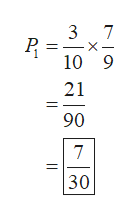A bag contains ten pencils. Three of the pencils are red, and the rest are brown. If two pencils are drawn successively at random and without replacement, find the following four probabilities: a) The first pencil-drawn is red and the second pencil-drawn is brown; b) Both pencils are red; c) One pencil is red and the other is brown (the order in which they are drawn doesn't matter); d) If the second pencil-drawn is red, what is the probability that the first pencil-drawn was

Question

A bag contains ten pencils. Three of the pencils are red, and the rest are brown. If two pencils are drawn successively at random and without replacement, find the following four probabilities: a) The first pencil-drawn is red and the second pencil-drawn is brown; b) Both pencils are red; c) One pencil is red and the other is brown (the order in which they are drawn doesn't matter); d) If the second pencil-drawn is red, what is the probability that the first pencil-drawn was

Step 1

Hello. Since your question has multiple sub-parts, we will solve first three sub-parts for you. If you want remaining sub-parts to be solved, then please resubmit the whole question and specify those sub-parts you want us to solve. Also, the fourth sub-part that is (d) is incomplete.

Step 2

Now, refer to the provided question:

Total number of pencils = 10

Number of red pencils = 3

Number of brown pencils = 7

(a)

The probability that the first pencil is red = 3/10

Now, there will be 9 pencil left without replacement,

so, the probability that the second pencil is brown = 7/9

Therefore,

The required probability that the first pencil is red and second is brown will be given by:help_outlineImage Transcriptionclose7 10 9 21 90 7 30 X fullscreen
Step 3

(b)

Now,

The probability that the first pencil is red = 3/10

Now, there will be total 9 pencil left and 2 red pencil left without replacement,

so, the probabil...

Want to see the full answer?

See Solution

Want to see this answer and more?

Our solutions are written by experts, many with advanced degrees, and available 24/7

See Solution
Tagged in

Basic Probability